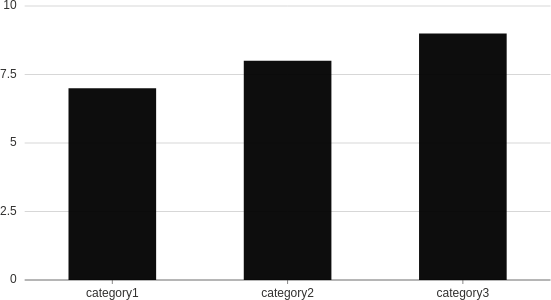# Visualization

Visualizations are charts/plots/graphs that help you better understand your data. Wave uses Grammar of Graphics to describe its plots.

For a more thorough explanation of how Wave plots work, please see Plotting.

Check the full API at ui.visualization.

## Basic visualization​``from h2o_wave import dataq.page['example'] = ui.form_card(box='1 1 4 4', items=[    ui.visualization(        plot=ui.plot([ui.mark(type='interval', x='=product', y='=price', y_min=0)]),        data=data(fields='product price', rows=[            ('category1', 7),            ('category2', 8),            ('category3', 9),        ]),    ),])``

## With dimensions​

In addition to the `width` attribute that is present on every form component, visualization also provides a way to control height via the `height` attribute, which defaults to `300px`. It supports all the CSS units, however, `%` may not always work as you could expect so we advise using static units like `px`, `rem` etc. instead.``from h2o_wave import dataq.page['example'] = ui.form_card(box='1 1 4 4', items=[    ui.visualization(        height='300px',        plot=ui.plot([ui.mark(type='interval', x='=product', y='=price', y_min=0)]),        data=data(fields='product price', rows=[            ('category1', 7),            ('category2', 8),            ('category3', 9),        ]),    ),])``

## With events​

Wave plots provide a way to listen for user clicks on certain parts of the visualization. All it takes is to register the event, e.g. `events=['select_marks']` and once the action happens, you get the appropriate data in `q.events.<name-attr>.select_marks`.``from h2o_wave import dataq.page['example'] = ui.form_card(box='1 1 4 4', items=[    ui.visualization(        name='viz',        height='300px',        plot=ui.plot([ui.mark(type='interval', x='=product', y='=price', y_min=0)]),        data=data(fields='product price', rows=[            ('category1', 7),            ('category2', 8),            ('category3', 9),        ]),        events=['select_marks'] # Submits q.events.viz.select_marks    ),])``